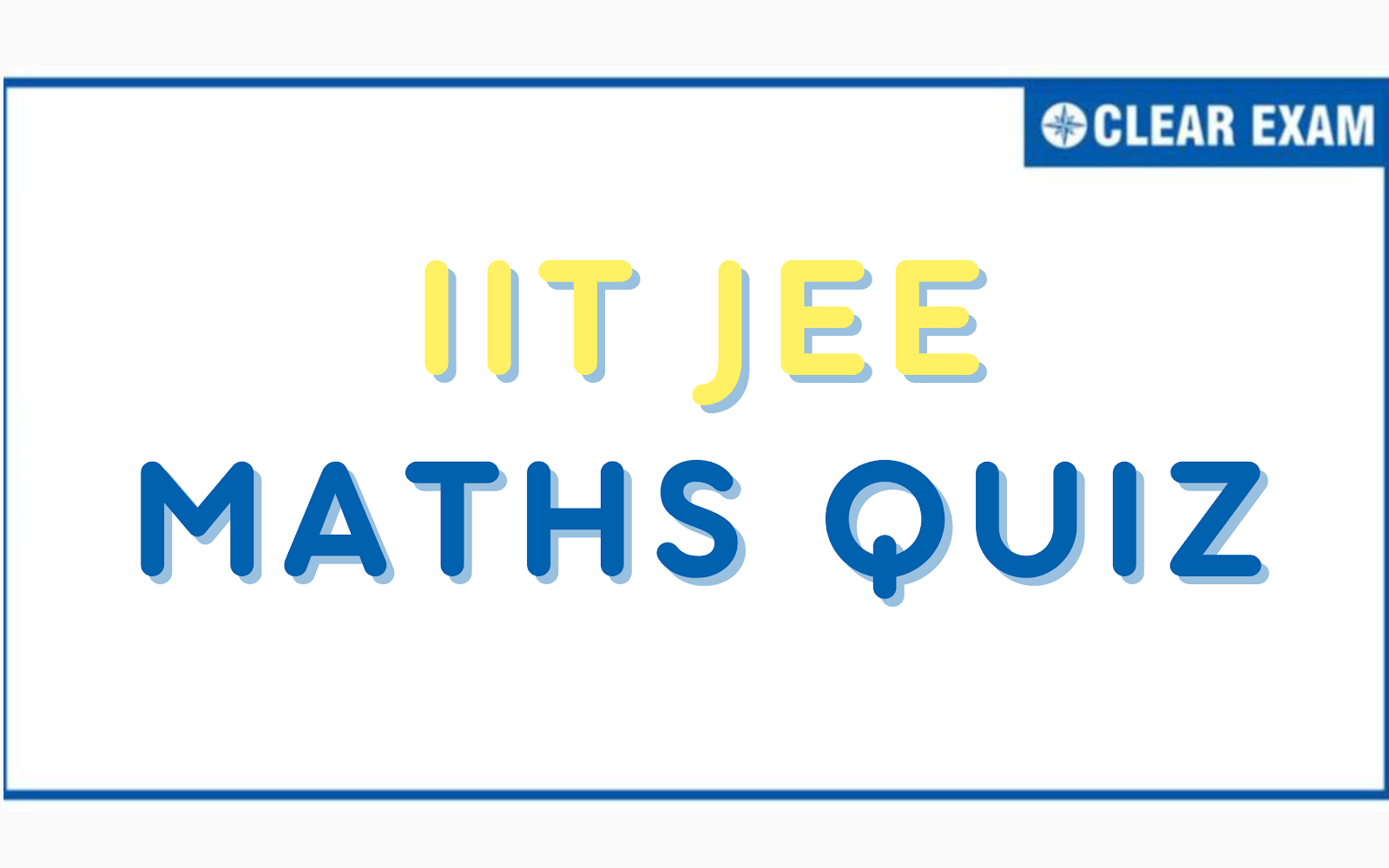## [LATEST]\$type=sticky\$show=home\$rm=0\$va=0\$count=4\$va=0CLEAR EXAM wants to be a single-stoppage highway, where every solution, of every question of top-notch reference books preferred by the schools can be available for the students. CLEAR EXAM provides you with a lot of IIT JEE Quiz Maths for prepration. IIT JEE Quiz Maths Practice for JEE Examination helps you to track JEE preparation progress and boost your score in JEE examination. It helps to improve your score by providing practice on important questions(Quiz) which are frequently asked in engineering entrance examinations such as JEE, BITSAT, VITEEE and state level engineering entrance examinations conducted earlier.

A highly competitive exam such as JEE demands speed and accuracy which primarily revolves around effective time management skills at the exam. This only comes with regular practice and incessant revisions. For this, we at CLEAR EXAM have collated a set of IIT JEE Quiz Maths on all JEE concepts which can be explored here.

IIT JEE has the following sections: Physics, Chemistry and Mathematics. Mathematics is such a subject which needs conceptual understanding. To do that, you have to practice a lot to remember all the formulae because these are very important to solve any problem. And when it comes to the IIT JEE exam, Maths holds sheer importance. Practising Maths alongside other subjects (Physics, Chemistry) as much as possible will help you to secure the best possible rank in the JEE Mains exam. This can be exercised here in the IIT JEE Quiz Maths section provided. The best strategy suggested by experts to tackle the JEE mathematics section is to adopt smart methodologies clubbed with diligence and dedication.

The concepts given below are some frequently asked topics in the JEE exam from the maths section. Get access to each topic and its Quizzes with just a click. We have provided Quizzes in a topic wise manner easily accessible by students for their convenience. The solutions are provided by our subject experts. We hope that our attempt of making IIT JEE Quiz Maths solutions available may prove to be helpful for the students

### IIT JEE Quiz Maths:

 Quiz on Fundamentals of Mathematics and Logarithm Quiz on Quadratic Equations Quiz on Trigonometric Ratios & Identities Quiz on Determinants Quiz on Matrix Quiz on Trigonometric Equations Quiz on Properties and Solutions of Triangle Quiz on Sets Quiz on Relations Quiz on Mathematical Reasoning Quiz on Principal of Mathematical Induction Quiz on Statistics Quiz on Functions Quiz on Inverse Trigonometric Functions Quiz on Limits Quiz on Continuity Quiz on Differentiability Quiz on Method of Differentiation Quiz on Straight Lines Quiz on Circle Quiz on Indefinite Integration Quiz on Definite Integration Quiz on Sequence and Series Quiz on Tangent and Normal Quiz on Monotonicity Quiz on Maxima-Minima Quiz on Area Under The Curve Quiz on Differential Equation Quiz on Parabola Quiz on Ellipse Quiz on Hyperbola Quiz on Vectors Quiz on 3D-Coordinate Geometry Quiz on Binomial Theorem Quiz on Complex Numbers Quiz on Permutation and Combination Quiz on Probability

## Want to know more

Please fill in the details below:

## Latest NEET Articles\$type=three\$c=3\$author=hide\$comment=hide\$rm=hide\$date=hide\$snippet=hide

Name

ltr
static_page
BEST NEET COACHING CENTER | BEST IIT JEE COACHING INSTITUTE | BEST NEET & IIT JEE COACHING: IIT JEE Quiz Maths
IIT JEE Quiz Maths
BEST NEET COACHING CENTER | BEST IIT JEE COACHING INSTITUTE | BEST NEET & IIT JEE COACHING
https://www.cleariitmedical.com/p/jee-quiz-maths.html
https://www.cleariitmedical.com/
https://www.cleariitmedical.com/
https://www.cleariitmedical.com/p/jee-quiz-maths.html
true
7783647550433378923
UTF-8

STAY CONNECTED